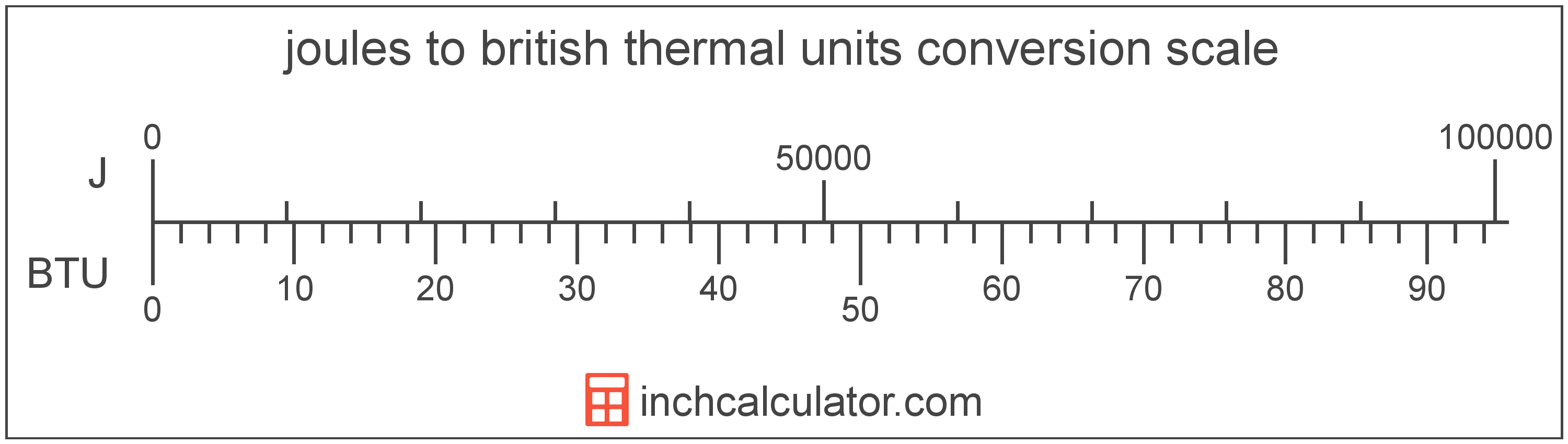# British Thermal Units to Joules Conversion

Enter the energy in british thermal units below to get the value converted to joules.

Results in Joules:1 BTU = 1,055.055853 J

## How to Convert British Thermal Units to JoulesTo convert a british thermal unit measurement to a joule measurement, multiply the energy by the conversion ratio.

Since one british thermal unit is equal to 1,055.055853 joules, you can use this simple formula to convert:

joules = british thermal units × 1,055.055853

The energy in joules is equal to the british thermal units multiplied by 1,055.055853.

For example, here's how to convert 5 british thermal units to joules using the formula above.
5 BTU = (5 × 1,055.055853) = 5,275.279263 J

## British Thermal Units

British thermal units are a measure of heat energy. Specifically, one british thermal unit is equal to the amount of heat energy required to increase the temperature of one pound of water one degree Fahrenheit. They are often used as a way to rate the energy heat producing appliances such furnaces, and to price heating fuels such as natural gas.

The british thermal unit is a US customary unit of energy. British thermal units can be abbreviated as BTU; for example, 1 british thermal unit can be written as 1 BTU.

## Joules

The joule is the energy equal to the force on an object of one newton at a distance of one meter. One joule is equal to the heat energy dissipated by the current of one ampere through one ohm of resistance for one second.

One joule is also equal to the energy needed to move an electric charge of one coulomb through a potential difference of one volt. In addition, one joule is also equal to the one watt-second.

The joule is the SI derived unit for energy in the metric system. Joules can be abbreviated as J; for example, 1 joule can be written as 1 J.

## British Thermal Unit to Joule Conversion Table

British thermal unit measurements converted to joules
British Thermal Units Joules
0.001 BTU 1.0551 J
0.002 BTU 2.1101 J
0.003 BTU 3.1652 J
0.004 BTU 4.2202 J
0.005 BTU 5.2753 J
0.006 BTU 6.3303 J
0.007 BTU 7.3854 J
0.008 BTU 8.4404 J
0.009 BTU 9.4955 J
0.01 BTU 10.55 J
0.02 BTU 21.1 J
0.03 BTU 31.65 J
0.04 BTU 42.2 J
0.05 BTU 52.75 J
0.06 BTU 63.3 J
0.07 BTU 73.85 J
0.08 BTU 84.4 J
0.09 BTU 94.96 J
0.1 BTU 105.51 J
0.2 BTU 211.01 J
0.3 BTU 316.52 J
0.4 BTU 422.02 J
0.5 BTU 527.53 J
0.6 BTU 633.03 J
0.7 BTU 738.54 J
0.8 BTU 844.04 J
0.9 BTU 949.55 J
1 BTU 1,055 J

## References

1. International Bureau of Weights and Measures, The International System of Units, 9th Edition, 2019, https://www.bipm.org/documents/20126/41483022/SI-Brochure-9-EN.pdf